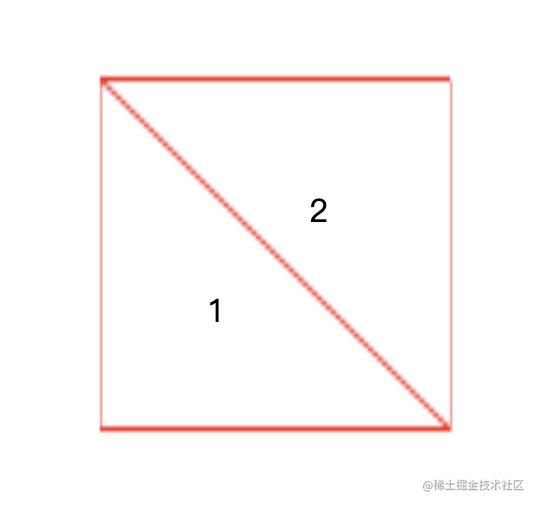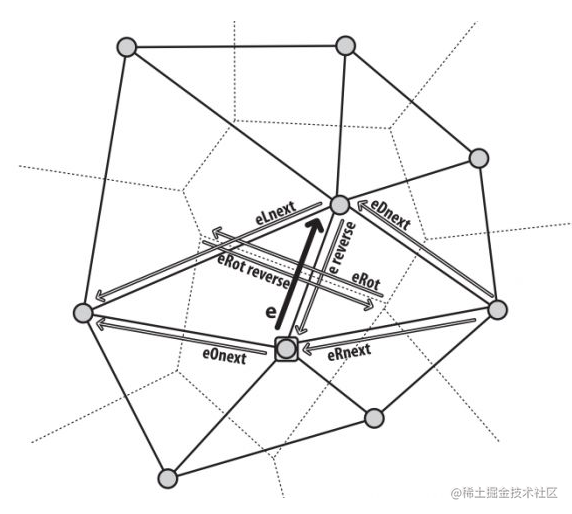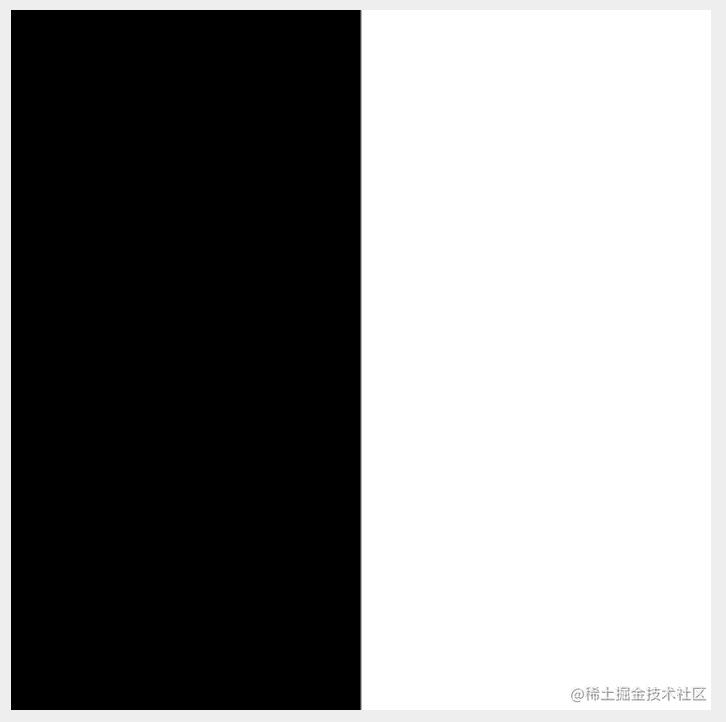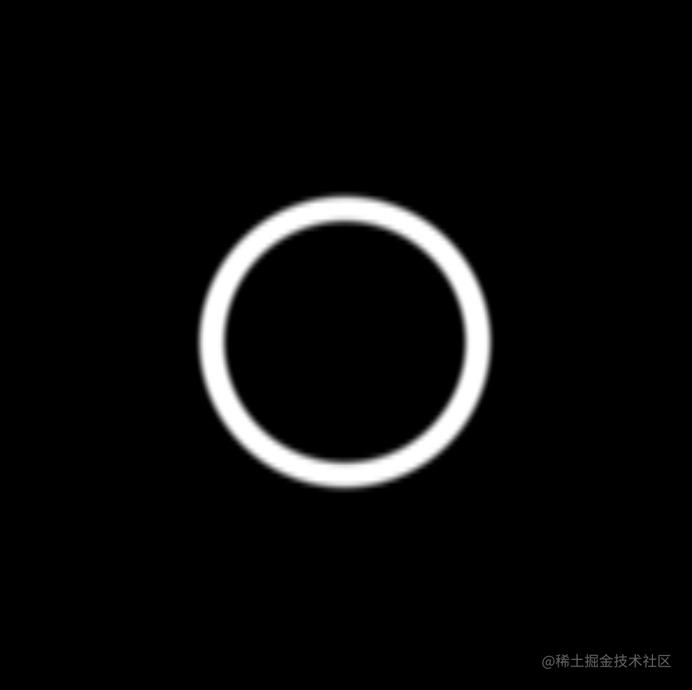# 【零基礎】充分理解WebGL（二）

```js renderer.setMeshData([ { positions: [[-1, -1, 0], [1, -1, 0], [1, 1, 0], [-1, 1, 0]], cells: [[0, 1, 3], [3, 1, 2]], }, ]);``````js const canvas = document.querySelector('canvas'); const renderer = new GlRenderer(canvas, {webgl2: true});

const fragment = ```#version 300 es precision highp float; out vec4 FragColor; void main() { FragColor = vec4(0, 0, 0, 1); }```; const program = renderer.compileSync(fragment); renderer.useProgram(program); renderer.render(); ```

```js const canvas = document.querySelector('canvas'); const renderer = new GlRenderer(canvas, {webgl2: true});

const fragment = ```#version 300 es precision highp float; out vec4 FragColor; uniform vec2 resolution; void main() { vec2 st = gl_FragCoord.xy / resolution; FragColor = vec4(0, 0, 0, 1); if(st.x > 0.5) { FragColor = vec4(1, 1, 1, 1); } }```; const program = renderer.compileSync(fragment); renderer.useProgram(program); renderer.uniforms.resolution = [canvas.width, canvas.height]; renderer.render(); ``````glsl

# version 300 es

precision highp float; out vec4 FragColor; uniform vec2 resolution; void main() { vec2 st = gl_FragCoord.xy / resolution; FragColor.rgb = step(0.5, st.x) * vec3(1.0); FragColor.a = 1.0; } ```

https://code.juejin.cn/pen/7100850170283163656

```glsl

# version 300 es

precision highp float; out vec4 FragColor; uniform vec2 resolution; void main() { vec2 st = gl_FragCoord.xy / resolution; vec2 center = vec2(0.5); FragColor.rgb = step(length(st - center), 0.2) * vec3(1.0); FragColor.a = 1.0; } ```

https://code.juejin.cn/pen/7100852428756484103

```glsl

# version 300 es

precision highp float; out vec4 FragColor; uniform vec2 resolution; void main() { vec2 st = gl_FragCoord.xy / resolution; vec2 center = vec2(0.5); float d = length(st - center); FragColor.rgb = smoothstep(d - 0.015, d, 0.2) * vec3(1.0); FragColor.a = 1.0; } ```

https://code.juejin.cn/pen/7100853943923638280

`smoothstep` 對階梯函式進行了平滑處理，它在範圍的上下限之間進行插值。

```glsl

# version 300 es

precision highp float; out vec4 FragColor; uniform vec2 resolution; void main() { vec2 st = gl_FragCoord.xy / resolution; vec2 center = vec2(0.5); float d = length(st - center); FragColor.rgb = (smoothstep(d - 0.015, d, 0.2) - smoothstep(d, d + 0.015, 0.18)) * vec3(1.0); FragColor.a = 1.0; } ``````glsl float stroke(float d, float d0, float w, float smth) { float th = 0.5 * w; smth = smth * w; float start = d0 - th; float end = d0 + th; return smoothstep(start, start + smth, d) - smoothstep(end - smth, end, d); }```

```glsl void main() { vec2 st = gl_FragCoord.xy / resolution; float d = stroke(st.x, 0.5, 0.02, 0.1); FragColor.rgb = d * vec3(1.0); FragColor.a = 1.0; }```

https://code.juejin.cn/pen/7100857056361447454

https://code.juejin.cn/pen/7100862005245902884